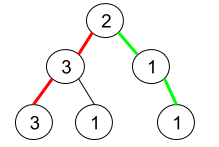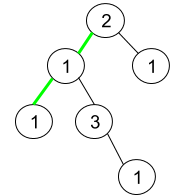Amazon

2020-05-23

## 1457. Pseudo-Palindromic Paths in a Binary Tree

### Question:

Given a binary tree where node values are digits from 1 to 9. A path in the binary tree is said to be pseudo-palindromic if at least one permutation of the node values in the path is a palindrome.

Return the number of pseudo-palindromic paths going from the root node to leaf nodes.

#### Example 1:``````Input: root = [2,3,1,3,1,null,1]
Output: 2
Explanation: The figure above represents the given binary tree. There are three paths going from the root node to leaf nodes: the red path [2,3,3], the green path [2,1,1], and the path [2,3,1]. Among these paths only red path and green path are pseudo-palindromic paths since the red path [2,3,3] can be rearranged in [3,2,3] (palindrome) and the green path [2,1,1] can be rearranged in [1,2,1] (palindrome).
``````

#### Example 2:``````Input: root = [2,1,1,1,3,null,null,null,null,null,1]
Output: 1
Explanation: The figure above represents the given binary tree. There are three paths going from the root node to leaf nodes: the green path [2,1,1], the path [2,1,3,1], and the path [2,1]. Among these paths only the green path is pseudo-palindromic since [2,1,1] can be rearranged in [1,2,1] (palindrome).
``````

#### Example 3:

``````Input: root = 
Output: 1
``````

Constraints:

• The given binary tree will have between `1` and `10^5` nodes.
• Node values are digits from `1` to `9`.

### Solution:

Using the similar idea as LeetCode Question 266, when finding the path to leaves by using DFS, decide if the permutaion of the path is palindrom.

``````/**
* Definition for a binary tree node.
* public class TreeNode {
*     int val;
*     TreeNode left;
*     TreeNode right;
*     TreeNode() {}
*     TreeNode(int val) { this.val = val; }
*     TreeNode(int val, TreeNode left, TreeNode right) {
*         this.val = val;
*         this.left = left;
*         this.right = right;
*     }
* }
*/
class Solution {
int counter = 0;
public int pseudoPalindromicPaths (TreeNode root) {
dfs(root, new int);
return counter;
}

private void dfs(TreeNode node, int[] hash) {
if(node == null) return;
hash[node.val]++;
if(node.left == null && node.right == null) {
if(isPalindrome(hash)) counter++;
hash[node.val]--;
return;
}
dfs(node.left, hash);
dfs(node.right, hash);
hash[node.val]--;
}

// Similar to Leetcode 266
private boolean isPalindrome(int[] hash) {
int oddcounter = 0;
for(int x : hash) {
if (x % 2 == 1){
oddcounter++;
}
if (oddcounter > 1) return false;
}
return true;
}
}
``````